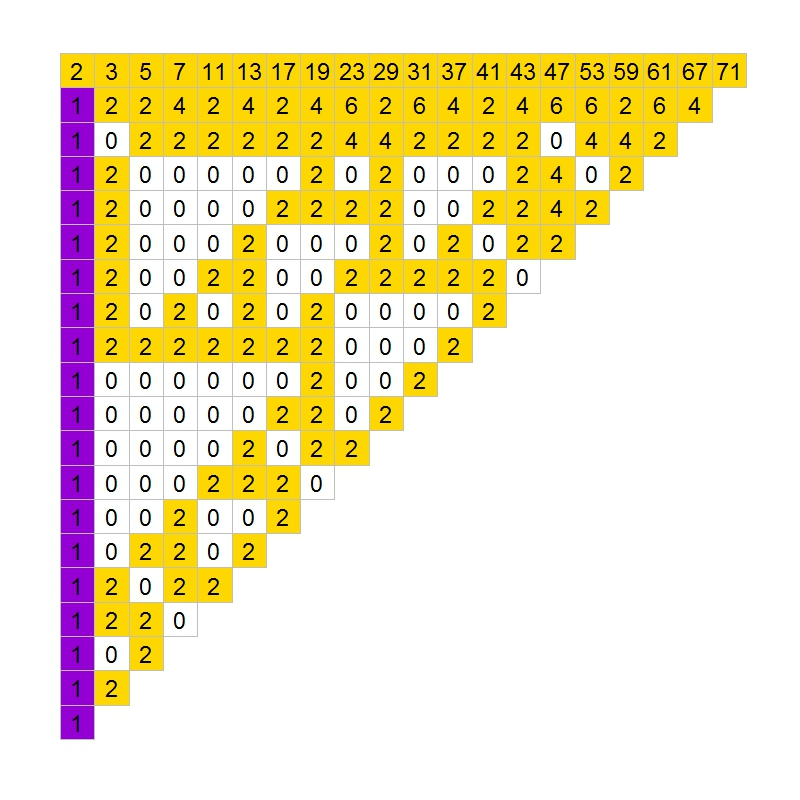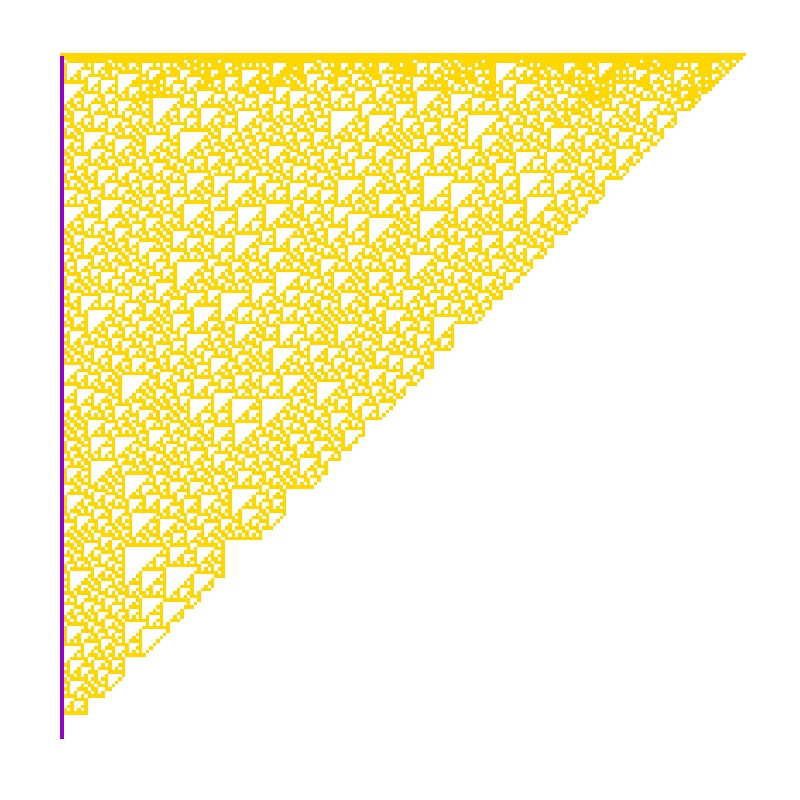# The Gilbreath’s Conjecture

317 is a prime, not because we think so, or because our minds are shaped in one way rather than another, but because it is so, because mathematical reality is built that way (G.H. Hardy)

In 1958, the mathematician and magician Norman L. Gilbreath presented a disconcerting hypothesis conceived in the back of a napkin. Gilbreath wrote first prime numbers in a row. In the next rows, he wrote the difference  in absolute value of consecutive values of previous row. Beginning with first 20 primes in the first row, he obtained something like this:The conjecture is easy: except for the first one, all elements of the first column are 1. So far, no one has demonstrated this hypothesis. In fact, according to mathematician Richard Guy, it seems unlikely that we will see a demonstration of Gilbreath’s conjecture in the near future, though probably this conjecture is true.

In the previous chart, I coloured zeros in white, ones in violet and rest of numbers in gold. The conjecture says that except for the first element, the first column is entirely violet. Following you can see the coloured chart for first 20, 40, 60 and 80 prime numbers:It is nice how zeros create triangular patterns similar to patterns created by cellular automata. This is the chart for first 200 primes:How much time will it take to demonstrate this simple conjecture? Who knows. Meanwhile, you can draw triangles with this code:

```library(ggplot2)
create.gilbreath=function(n)
{
require(reshape)
require(schoolmath)
data(primlist)
gilbreath=t(matrix(primlist[2:(n+1)], n, n))
for (i in 2:n) {gilbreath[i,]=c(eval(parse(text=paste(c(paste("abs(diff(",collapse=""), "gilbreath[i-1,]", paste("))",collapse="")),collapse=""))),rep(NA,1))}
na.omit(melt(t(gilbreath)))
}
opt=theme(legend.position="none",
panel.background = element_blank(),
panel.grid = element_blank(),
axis.ticks=element_blank(),
axis.title=element_blank(),
axis.text =element_blank())
gilbreath=create.gilbreath(20)
gilbreath\$value1=cut(gilbreath\$value, breaks=c(-Inf,1:2,Inf), right = FALSE)
ggplot(gilbreath, aes(x=X1, y=X2)) +
geom_tile(aes(fill = value1), colour="grey") +
scale_fill_manual(values = c("white", "darkviolet", "gold"))+
geom_text(label=gilbreath\$value, size=8)+
scale_y_reverse()+opt
```

## 9 thoughts on “The Gilbreath’s Conjecture”

1.ajdamico says:

this is beautiful, thank you

2.vzemlys says:

I do not understand why did you use parse? Why not simply write the exact code?:
for(i in 2:n) gilbreath[i,] <- c(abs(diff(gilbreath[i-1,])),NA)

Also geom_text is probably unnecessary. Otherwise, nice post.

1.aschinchon says:

You are right, thanks. My first approach was another in which I used parse to evaluate expressions on the fly. I changed it but I reused the original code so this is why still appears parse function. I used geom_text to show numbers in the first chart. I don’t use it for the rest of charts. thanks for your comment!

3.claudio says:

Hi, just question: this is not working for me:

opt <- theme(legend.position=”none”,

Something with opt &lt, maybe?

1.aschinchon says:

Solved. WordPress translates <- into &lt and sometimes I don't remember it. Thanks!

4.claudio says:

I know it’s laziness from me…but how do you generate the amazing: last picture?

1.aschinchon says:

try this:

gilbreath=create.gilbreath(200)
gilbreath\$value1 <- cut(gilbreath\$value, breaks=c(-Inf,1:2,Inf), right = FALSE)
ggplot(gilbreath, aes(x=X1, y=X2)) +
geom_tile(aes(fill = value1)) +
scale_fill_manual(values = c("white", "darkviolet", "gold"))+
scale_y_reverse()+opt

5.Hans W Borchers says:

Please note that the ‘schoolmath’ package is known to be a mess and should
— for quality reasons — not be listed on CRAN.
For example, the primes() function returns for the range 1 to 200:

schoolmath::primes(1, 200)
 1 2 3 5 7 11 13 17 19 23 29 31
 37 41 43 47 53 59 61 67 71 73 79 83
 89 97 101 103 107 109 113 127 131 133 137 139
 149 151 157 163 167 173 179 181 191 193 197 199

of which 1 (of course) and 133 = 7 * 19 are not primes.This continues into higher ranges, sometimes forgetting primes, sometimes including non-primes.
In packages ‘matlab’ or ‘pracma’ you will find correct primes() functions.

1.aschinchon says:

Thank you very much! I will change the package.